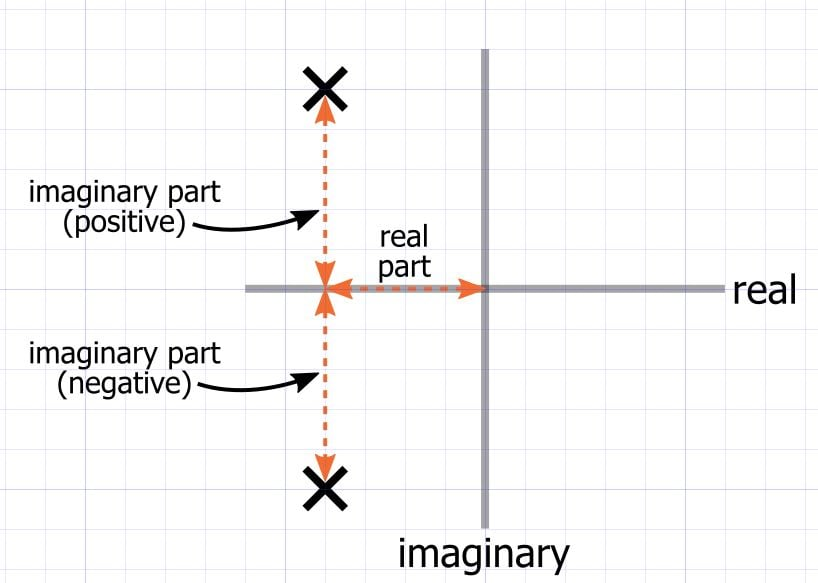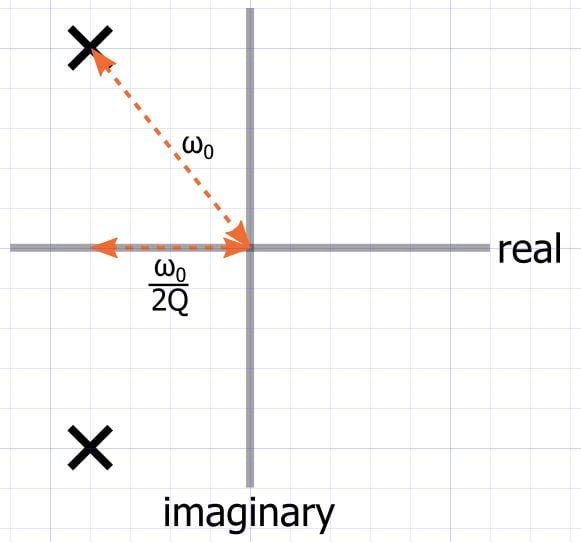Technical Article

# Understanding Complex-Conjugate Poles in Filter Theory

August 30, 2016 by Robert Keim

## This technical brief explains the importance of complex-conjugate poles and second-order stages in optimizing filter performance.

This technical brief explains the importance of complex-conjugate poles and second-order stages in optimizing filter performance.

### Related Information

There are two ways to achieve second-order (i.e., two-pole) filter response: cascade two first-order filters, or use a second-order topology. An example of the former is two resistor–capacitor (RC) low-pass filters connected in series, with the output of the first buffered by a voltage follower. Examples of the latter are passive resistor–capacitor–inductor (RLC) filters and active filters, such as the Sallen­–Key.

Of course, this discussion applies also to higher-order filters: a four-pole response can be provided by four cascaded first-order stages or two cascaded second-order stages.

There is an appealing simplicity surrounding the cascade-first-order-stages approach. All you need for a second-order filter is some rudimentary math, an op-amp, two resistors, and two capacitors (three if you include the op-amp’s bypass cap). Why so much ado about second-order topologies, then? Well, the answer to that question leads to an important concept in filter theory: complex-conjugate poles.

Recall that complex conjugates have real parts that are equal in magnitude and sign and imaginary parts that are equal in magnitude and opposite in sign. Let’s visualize this using the s-plane:Here we have complex-conjugate poles on the left side of the imaginary axis (which is where you want the poles, unless you’re designing an oscillator instead of a filter). They have equal distance from the real axis and the imaginary axis, but they are mirrored across the real axis because one has a positive imaginary part and one has a negative imaginary part.

Complex-conjugate poles are important because they allow the designer to optimize a filter such that it exhibits a maximally flat passband, a rapid transition from passband to stopband, or constant group delay (i.e., linear phase response). The problem with cascaded first-order stages is that this configuration cannot provide complex-conjugate poles.

Let’s explore this fact using a unity-gain low-pass filter as an example. The s-domain transfer function is

$$H(s)=\frac{1}{s+\omega_0}$$

Cascading two of these filters corresponds to multiplying the two transfer functions:

$$H(s)=\frac{1}{s+\omega_0}\times\frac{1}{s+\omega_0}=\frac{1}{s^2+2\omega_0 s+\omega_0^2}$$

The term we are interested in here is the 2ω0s. The denominator of a generalized second-order transfer function can be written as

$$s^2+\frac{\omega_0}{Q} s+\omega_0^2$$

Thus, we have

$$\frac{\omega_0}{Q}=2\omega_0 \ \Rightarrow \ Q = 0.5$$

The first thing to notice here is that the Q factor cannot be adjusted so as to fine-tune the frequency response. Two cascaded first-order filters will always have Q = 0.5 (furthermore, Q = 0.5 corresponds to a rather gradual transition from passband to stopband and significant attenuation in the passband).

The second thing to understand is that you cannot have complex-conjugate poles when Q is 0.5. Consider the following diagram:The distance from the imaginary axis to a pole is equal to ω0/2Q, and the distance from the origin to a pole is ω00 is the pole frequency). If Q = 0.5, we have ω0/(2 × 0.5) = ω0, and thus the distance from the imaginary axis will be equal to the distance from the origin. It follows that the pole must be located on the real axis, and consequently there is no possibility for a complex-conjugate pair because the pole location has no imaginary part.

Perhaps we can intuitively conclude from the circuit implementation that cascaded-first-order-stage filters do not allow for optimization. But it is helpful to recognize that this rigidity is bound to the absence of complex-conjugate poles, which can be produced using a true second-order stage and which enable the designer to optimize a filter for a particular application.

1 Comment•victor acuña December 21, 2017

Thanks for the article, it helps me understand the one pole filter quality factor.

Like.